## 3.5 Universality, again

Although this may all seem tediously abstract, it is surprisingly useful. Take another look at the single-qubit interference circuit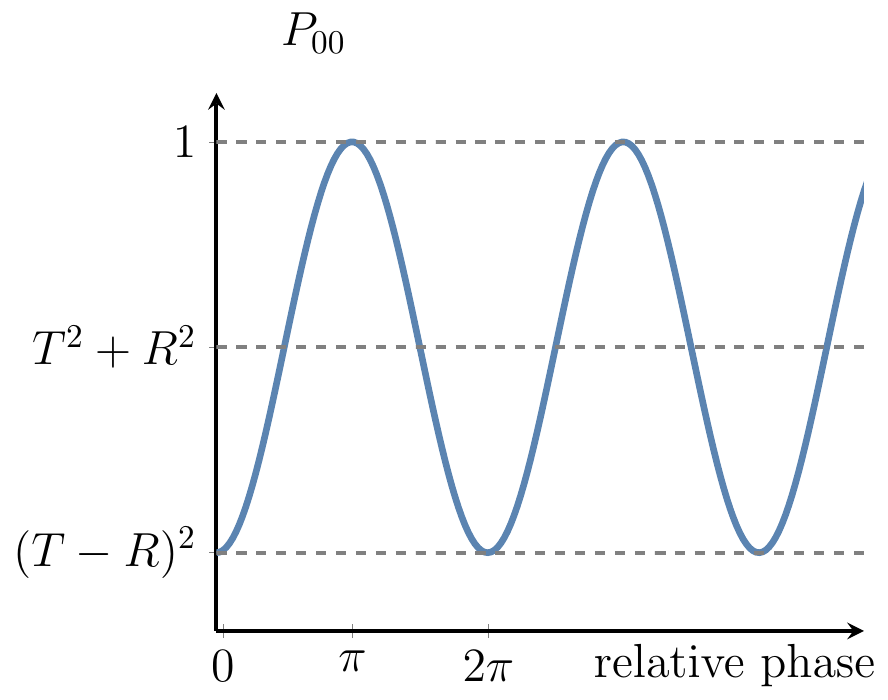and the corresponding sequence of unitary operations \begin{aligned} H \left( e^{-i\frac{\varphi}{2}Z} \right) H &= e^{-i\frac{\varphi}{2}X} \\&= \begin{bmatrix} \cos\varphi/2 & -i\sin\varphi/2 \\-i\sin\varphi/2 & \cos\varphi/2 \end{bmatrix} \end{aligned}

The single-qubit interference circuit has a simple geometrical meaning: it shows how a rotation about the z-axis, induced by the phase gate P_\varphi, is turned, by the two Hadamard gates, into a rotation about the x-axis.

Now, take a look at this circuit: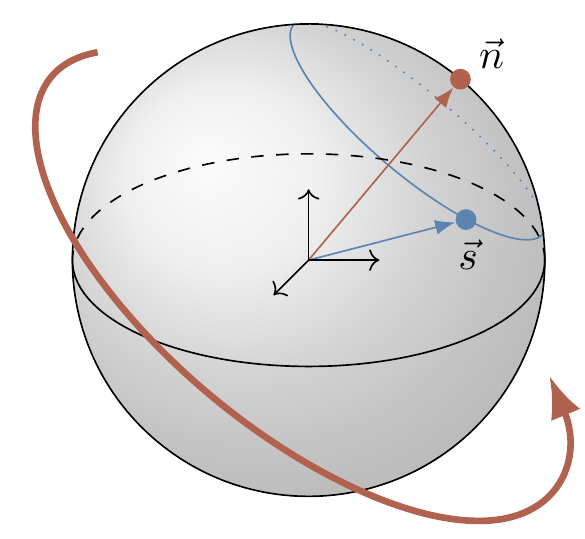What does it represent? The central part is a rotation by \varphi about the x-axis, sandwiched between two rotations about the z-axis. Recall our previous discussion (Section 2.12) about a universal set of gates: any rotation in the Euclidean space can be performed as a sequence of three rotations: one about z-axis, one about x-axis, and one more about the z-axis. In this context, this implies that any unitary U, up to a global phase factor, can be written as \begin{aligned} U(\alpha, \beta, \varphi) &= e^{-i\frac{\beta}{2}Z} e^{-i\frac{\varphi}{2}X} e^{-i\frac{\alpha}{2}Z} \\&= \begin{bmatrix} e^{-i\left(\frac{\alpha+\beta}{2}\right)}\cos\frac{\varphi}{2} & ie^{i\left(\frac{\alpha-\beta}{2}\right)}\sin\frac\varphi{2} \\ie^{-i\left(\frac{\alpha-\beta}{2}\right)}\sin\frac\varphi{2} & e^{i\left(\frac{\alpha+\beta}{2}\right)}\cos\frac\varphi{2} \end{bmatrix}. \end{aligned}

That is, once you are given a pair of Hadamard gates and an infinite supply of phase gates (so that you can choose the three phases you need) you can construct an arbitrary unitary operation on a single qubit.

It is important to note that the two axes in question, z and x, do not have any special status, geometrically speaking — if we have rotations about any two orthogonal80 axes then we can create any one-qubit unitary that we want.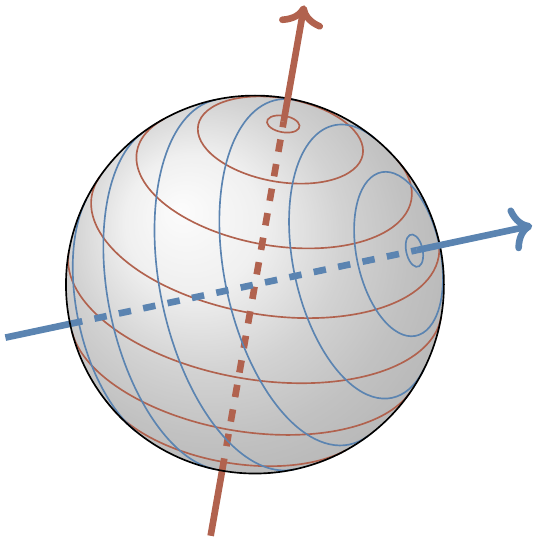Figure 3.8: If we can move along the two families of circles, then from any point on the sphere we can reach any other point. The two axes do not even have to be orthogonal: any two different (i.e. non-collinear) axes will do! Can you see why?

Now consider the following circuit: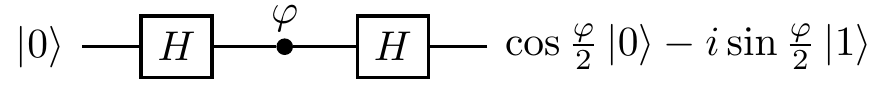where both A and B are unitary operations. We claim that any unitary U can be represented in this form, for some A and B.

Again, we can prove this geometrically. The circuit represents two rotations by 180^\circ about the two axes obtained by rotating the z-axis via unitaries A and B, respectively. Any rotation in the three-dimensional space is the composition of two rotations by 180^\circ, as shown in Figure 3.9. The resulting axis of rotation is perpendicular to the two axes about which rotations by 180^\circ are performed, and the angle of the composed rotation is twice the angle between the two axes.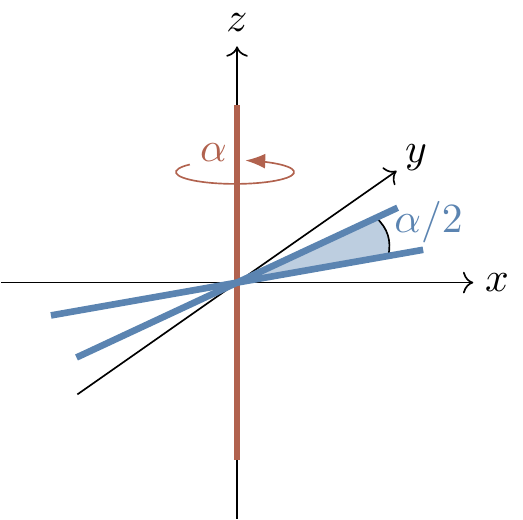Figure 3.9: Rotating by \alpha around the z-axis is the same as the composition of two rotations by 180^\circ around axes which both lie in the xy-plane, with angle \alpha/2 between them.

1. In fact, even this orthogonality condition isn’t necessary! See Figure 3.8↩︎